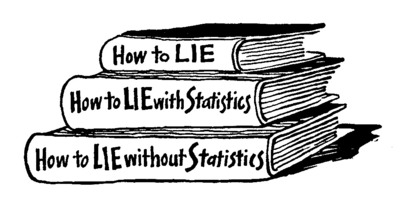Intro Research PhD study R codes Teaching Extra

## NMST434 Modern statistical methods (official information)

Exercise class: Thursday 14:00 - 15:30 seminar room of the Department of Probability and Math. Statistics (S. Nagy)

Lecture material and actual information

Classroom teaching has been cancelled until further notice. For the duration of the closure, this course will be taught by distant education methods. Questions and requests for clarification can be asked by phone or email.

The lecture will proceed by the self-study. The preliminary plan is as follows:

Assumed knowledge:

It is assumed that the students have already a very solid knowledge of statistics and probability theory. This is covered by the following courses Mathematical Statistics 1 and 2 (NMSA331 and NMSA332), Probability Theory 1 (NMSA333) and Linear regression (NMSA407).

The student who have not passed these courses (e.g. students coming within the Erasmus+ programme) should check if they are familiar with the theory covered in:
Mukhopadhyay, N. (2000). Probability and statistical inference. CRC Press (the whole book except for Chapters 10 and 13);
Khuri, A. I. (2009). Linear model methodology. Chapman and Hall/CRC (Chapters 1 - 6).